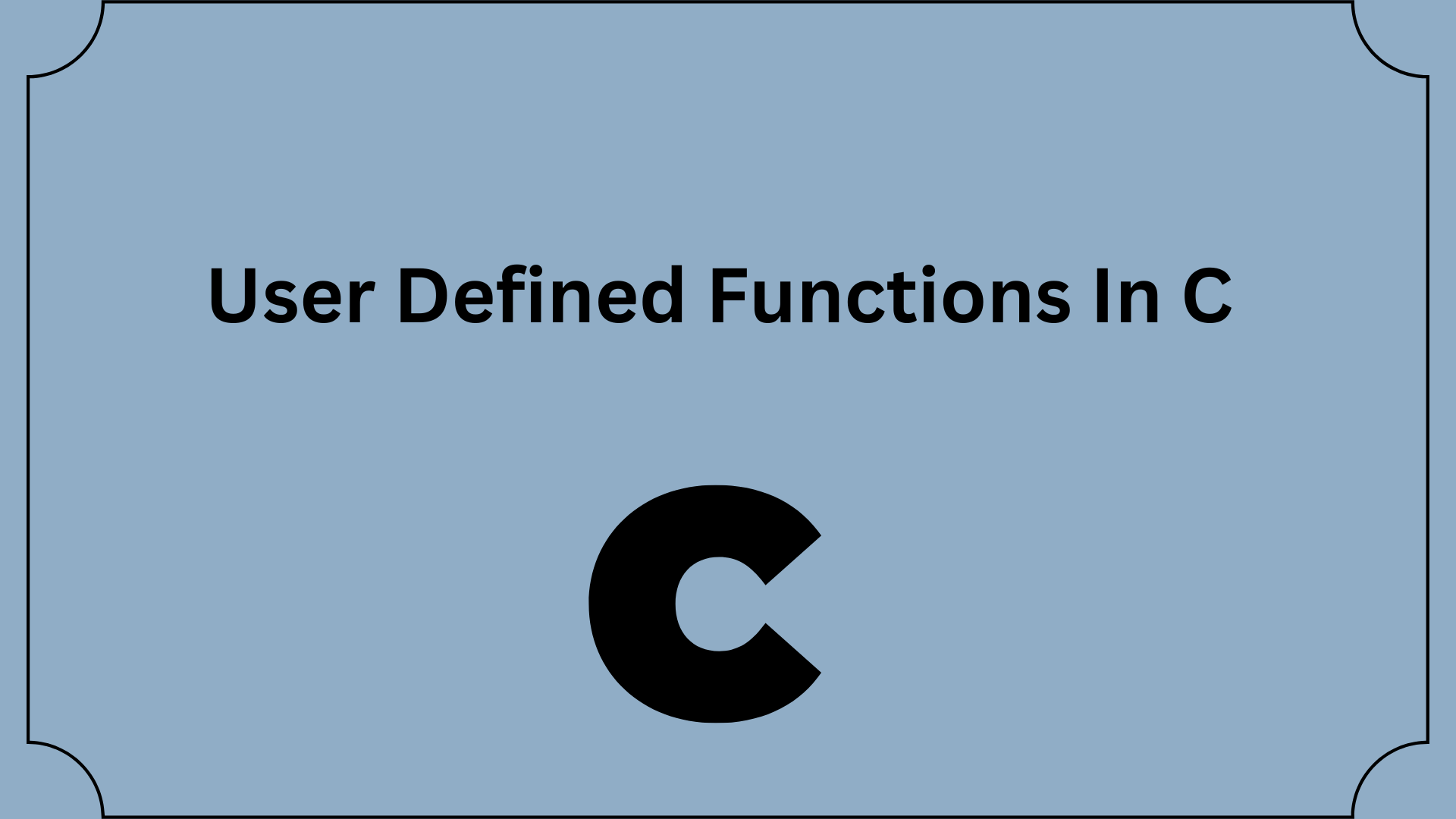## User Defined Functions In C Programming Language

With the aid of an example, you will learn how to write user-defined functions in C programming in this article.

A unit of code known as a function carries out a particular task.

Call by value or call by reference are two ways to call additional functions. In order to improve code reuse and make it easier to test, debug, and maintain the code, functions must only be created once and can be called as many times as necessary inside the program.

A chunk of code known as a function can be used to carry out a particular activity. User-defined functions, often known as custom functions, are supported in C. Function declarations, definitions, and calls are the three primary parts of a user-defined function.

• User-defined functions are defined in this article along with a description of their use in programs.
• The declaration and definition of a user-defined function in C programs are covered in this article.
• This article also discusses how user-defined functions are called in C applications.
• The handling of arrays and other types of data structures in functions is not covered in this article.

You can define functions in C based on your needs. These processes are referred to as user-defined processes. For instance:

You need to draw a circle and give it a specific color based on its radius. Two functions can be developed to address this issue:

• the function create circle()
• function color()

Contents

## Example: User-defined function in c

Using two integers to add is demonstrated here. To complete this task, we developed a user-defined add numbers function ().

```#include <stdio.h>
int addNumbers(int a, int b);         // function prototype

int main()
{
int n1,n2,sum;

printf("Enters two numbers: ");
scanf("%d %d",&n1,&n2);

sum = addNumbers(n1, n2);        // function call
printf("sum = %d",sum);

return 0;
}

int addNumbers(int a, int b)         // function definition
{
int result;
result = a+b;
return result;                  // return statement
}```

## A prototype for a function(User Defined Functions In C)

The declaration of a function, which includes the name, parameters, and return type, is known as a function prototype. It is devoid of the function body.

The compiler is informed by a function prototype that the function might eventually be used in the program.

## Syntax of function prototype( User Defined Functions In C )

`returnType functionName(type1 argument1, type2 argument2, ...);`

The function prototype in the above example, int add numbers(int a, int b), gives the compiler the following information:

• The function’s name is add number ()
• The function’s return type is int.
• The function receives two inputs of type int.

If the user-defined function is defined before the main() function, the function prototype is not required.

## making a function call(User Defined Functions In C )

By invoking a user-defined function, control of the program is handed to that function.

## Syntax of a function call(User Defined Functions In C)

`functionName(argument1, argument2, ...);`

The addnumber(n1, n2); statement in the main() function is used in the example above to invoke the function.

## Function definition

The code blocks needed to do a specific task are contained in the function definition. In our example, two numbers are added and then returned.

The definition of a function in syntax

```returnType functionName(type1 argument1, type2 argument2, ...)
{
//body of the function
};```

The program’s control is transferred to the function definition when a function is invoked. And the compiler begins running the programs included within a function’s body.

## giving a function arguments

In computer programming, an argument is a variable that is supplied to a function. Two variables, n1 and n2, are provided to the function call in the example above.

In the definition of the function, the parameters a and b take the passed arguments. These are referred to as the function’s formal parameters.

The formal parameters and the arguments given to a function must be of the same type in order for the compiler to avoid throwing an error.

A should also be of the char type if n1 is of the char type. Variable b should also be of the float type if n2 is.

It’s also possible to invoke a function without providing any arguments.

## Return Statement

A function’s execution is stopped and a value is returned to the caller function by the return statement. After the return statement, control of the program is handed over to the caller function.

The main function receives the value of the result variable in the aforementioned example. This value is passed to the main() function’s sum variable.

## Return statement syntax

`return (expression); `

For example,

```return a;
return (a+b);```

The type of value returned from the function and the return type specified in the function prototype and function definition must match.